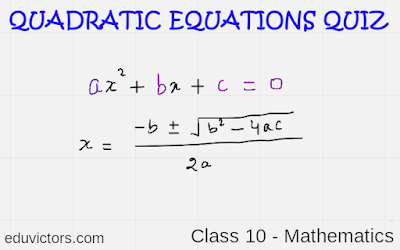# Class 10 Mathematics -  Quadratic Equations Quiz

Choose the correct option from the given choices in each question.

Q1.  The quadratic equation ax2 + bx + c = 0 will have zero number of real zeroes if-

1. b2 > 4ac
2. b2 = 4ac
3. b2 < 4ac
4. none of these
Q2. A quadratic equation ax2 + bx + c = 0 with real roots can have-
1. two linear factors
2. one linear factor
3. three linear factors
4. four linear factors

Q3. Which of the following forms of quadratic equations given below will give the same roots as given by the quadratic equation ax2 + bx + c = 0.
1. (ax - α)(x - β) = 0, where α and β are roots of the equation ax2 + bx + c = 0.
2. (x - α)(ax - β) = 0, where α and β are roots of the equation ax2 + bx + c = 0.
3. (x - α)(x - β) = 0, where α and β are roots of the equation ax2 + bx + c = 0.
4. (ax - α)(ax - β) = 0, where α and β are roots of the equation ax2 + bx + c = 0.

Q4. Which of the following statement is true?
1. every quadratic equation has two distinct linear factors
2. every quadratic equation has distinct linear factors
3. every quadratic equation has two same linear factors
4. every quadratic equation has at most two linear factors

Q5. If the quadratic equation has equal zeroes then which of the statement is true?
1. linear factors are identical
2. linear factors are distinct
3. depends on the coefficients of the equation
4. none of these

Q6.  In the quadratic equation ax2 + bx + c = 0 where a, b and c are rational numbers if one of the root is x = 2 + √3 the other will be-
1. depends on a, b and c.
2. x = 2 + √3
3. x = 2 - √3
4. x = 3 - √2

Q7. One factor of the quadratic equation ax2 + bx + c = 0 is (x - 5 + √2) other factor will be-
1. depends upon a, b and c.
3. (x - 2 + √5)
4. (x + 5 - √2)

Q8. The quadratic equation whose factors are x = -a, a/2 is-
1. 22 + ax + a2 = 0
2. x2 + ax - a2 = 0
3. 2x2 + ax - a2 = 0
4. 2x2 + x - a2 = 0

1: c. b2 < 4ac
2: a. a two linear factors
3: c. (x - α)(x - β) = 0, where α and β are roots of the equation ax2 + bx + c = 0.
4: d. every quadratic equation has at most two linear factors
5: a. linear factors are identical
6: c. x = 2 - √3
7: a. depends upon a, b and c. (depends upon a, b and c whether they are irrational or rational)
8: c. 2x2 + ax - a2 = 0